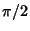## Right Angle

An Angle equal to half the Angle from one end of a line segment to the other. A right angle isradians or 90°. A Triangle containing a right angle is called a Right Triangle. However, a Triangle cannot contain more than one right angle, since the sum of the two right angles plus the third angle would exceed the 180° total possessed by a Triangle.

See also Acute Angle, Oblique Angle, Obtuse Angle, Right Triangle, Semicircle, Straight Angle, Thales' Theorem